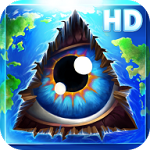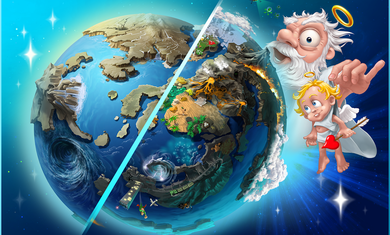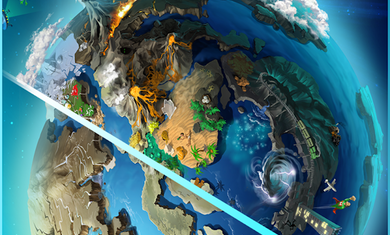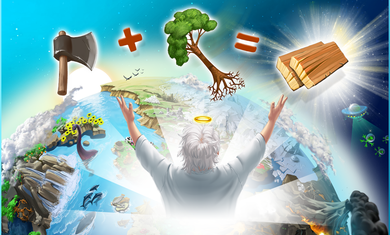6188手游网 > 手机游戏 > 休闲益智 > 涂鸦上帝中文版# 涂鸦上帝中文版

• 类型：休闲益智
• 版本：v3.2.5
• 语言：简体中文
• 大小：67MB
• 等级：
• 更新：2020-01-22• 游戏介绍
• 相关游戏
• 相关文章

•••涂鸦上帝合成表：

1、风

风+土=尘土 无线电波+无线电波=放射性 能量+风=风暴 火+尘土=灰烬 真空+电=无线电波 岩浆+水=石头+蒸汽

2、火

土+火=岩浆 能量+火=等离子体 细菌+沼泽=虫子+硫磺 树+火=煤炭 煤炭+水=石油 化肥+石灰石=硝石 等离子体+虚空=太阳

3、水

酒精+水=伏加特 火+水=酒精 水+金属=快银 土+水=沼泽

4、土

沙+火=玻璃 放射性+金属=钚 草+家畜=奶+肥料 金属+贤者之石=黄金 沙+沼泽=粘土 火+石头=金属 风+石头=沙 贝壳+石头=石灰石

岩浆+水=石头+蒸汽

5、八卦图

石头+生命=蛋 金属+能量=电 工具+蝎子=毒=蘑菇+工具 风+水=能量=酒精+火 能量+沼泽=生命 半神+快银=贤者之石

人+人=性 虚空=创造100个元素后系统赠送的 猎人+恐龙=恐龙+血 沙+生命=种子6、骷髅头

火+鸟=凤凰 灰烬+生命=鬼魂 生命+尸体=僵尸 吸血鬼+野兽=狼人 风暴+鸟=雷鸟 恐龙+火=龙 粘土+生命=泥人

尸体+僵尸=食尸鬼 树+生命=树人

7、动物(猫咪图标)

水+野兽=鲸鱼 蛋+土=恐龙 风+蛋=鸟 蛋+沙=乌龟 蜥蜴+土=野兽 水+蛇=鱼 人+野兽=家畜 野兽+鱼=海豚 沼泽+虫=蛇

8、植物

土+苔藓=草 太阳+草=花 沼泽+苔藓=蕨类 种子+能量=咖啡 沼泽+草=芦苇 花+树=苹果 种子+土=树 太阳+花=向日葵

草+火=烟草 沙+树=棕榈

9、虫子、微生物类

浮游生物+石头=贝壳 细菌+水=浮游生物 虫子+风=蝴蝶 虫子+土=甲虫 土+杂草=蘑菇 沼泽+杂草=苔藓 生命+沼泽=细菌

水+生命=杂草 浮游生物+土=虫子 沙+甲虫=蝎子

10、基本生活用品

人+家畜=毛+奶+肉 火+生面团=面包 石头+小麦=面粉 猎人+鸟=血+羽毛+肉 水+面粉=生面团 纸+烟草=香烟 种子+田地=小麦

小麦+酒精=啤酒 猎人+野兽=肉+毛+血

11、科技

武器+毒=涂毒武器 混凝土+砖头=房子 人+金属=工具 蒸汽+金属=锅炉 硫磺+硝石=火药 房子+玻璃=摩天大楼 人+石头=棚屋

武器+火药=枪械 工具+金属=武器 煤炭+锅炉=蒸汽机

12、人

贤者之石+人类=半神 巫师+能量=半神 涂毒武器+人=刺客 人+伏加特=酒鬼=啤酒+人类 人+武器=猎人

生命+野兽=人 人+电脑=生化机器人 人+火=尸体=人+毒药 人+能量=巫师 龙+战士=英雄+血 武器+猎人=战士13、交通(汽车)

小船+木头=大船 大船+纺织物=帆船 风+汽车=飞机 拖车+蒸汽机=火车头 工具+木头=轮子 拖车+家畜=马车

拖车+石油=汽车 木头+轮子=拖车 水+木头=小船 蒸汽机+大船=蒸汽轮船

14、文明(金字塔和面具)

工具+毛=纺织物 水+水泥=混凝土 树+工具=木头 纸+羽毛=书 石灰石+粘土=水泥 人类+粘土=陶瓷 工具+土=田地

人+纺织物=衣服 工具+芦苇=纸 粘土+火=砖头

15、高科技

激光+书=唱片 虚空+玻璃=灯泡 电视+书=电脑 无线电波+灯泡=电视 钚+武器=核弹 电脑+电脑=互联网 虚空+飞机=火箭

无线电波+火=激光 无线电波+电脑=手机 虚空+火箭=卫星涂鸦上帝中文版游戏特色：

1、该游戏是一款全新的幸存者任务，在逃离大洋中的神秘岛屿，共有3种不同的结局，你的结局会是什么?

2、全新的20世纪最伟大的发明，20世纪中每十年的发明，全新“谜题” 模式。你能找到最后的目标吗。

3、创造 300 多个高级物品和理念，直觉式的游戏玩法，鼓励玩家带着思考进行创造性的游戏。

4、混合火、水、土和空气等元素，创造火车、摩天大楼以及更多，创造宇宙。

特别说明

涂鸦上帝破解版，修改闪电数量无限，完美汉化游戏内容，去除广告，解锁付费游戏免费下载。

相关文章

换一换